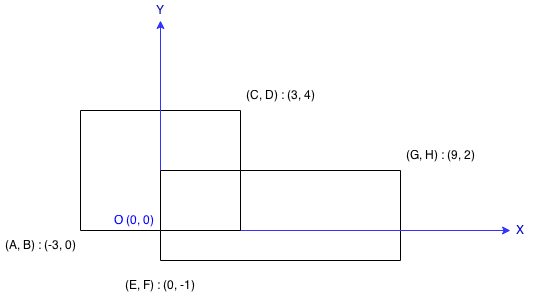223. Rectangle Area

Find the total area covered by two rectilinear rectangles in a 2D plane.

Each rectangle is defined by its bottom left corner and top right corner as shown in the figure.Example:

```Input: A = -3, B = 0, C = 3, D = 4, E = 0, F = -1, G = 9, H = 2
Output: 45```

Note:

Assume that the total area is never beyond the maximum possible value of int.

223. Rectangle Area
``````struct Solution;

impl Solution {
fn compute_area(a: i32, b: i32, c: i32, d: i32, e: i32, f: i32, g: i32, h: i32) -> i32 {
let left = a.max(e);
let right = c.min(g);
let bottom = b.max(f);
let top = d.min(h);
let r1 = (c - a) * (d - b);
let r2 = (g - e) * (h - f);
let r3 = (right.max(left) - left) * (top.max(bottom) - bottom);
r1 + r2 - r3
}
}

#[test]
fn test() {
let a = -3;
let b = 0;
let c = 3;
let d = 4;
let e = 0;
let f = -1;
let g = 9;
let h = 2;
let res = 45;
assert_eq!(Solution::compute_area(a, b, c, d, e, f, g, h), res);
}
``````